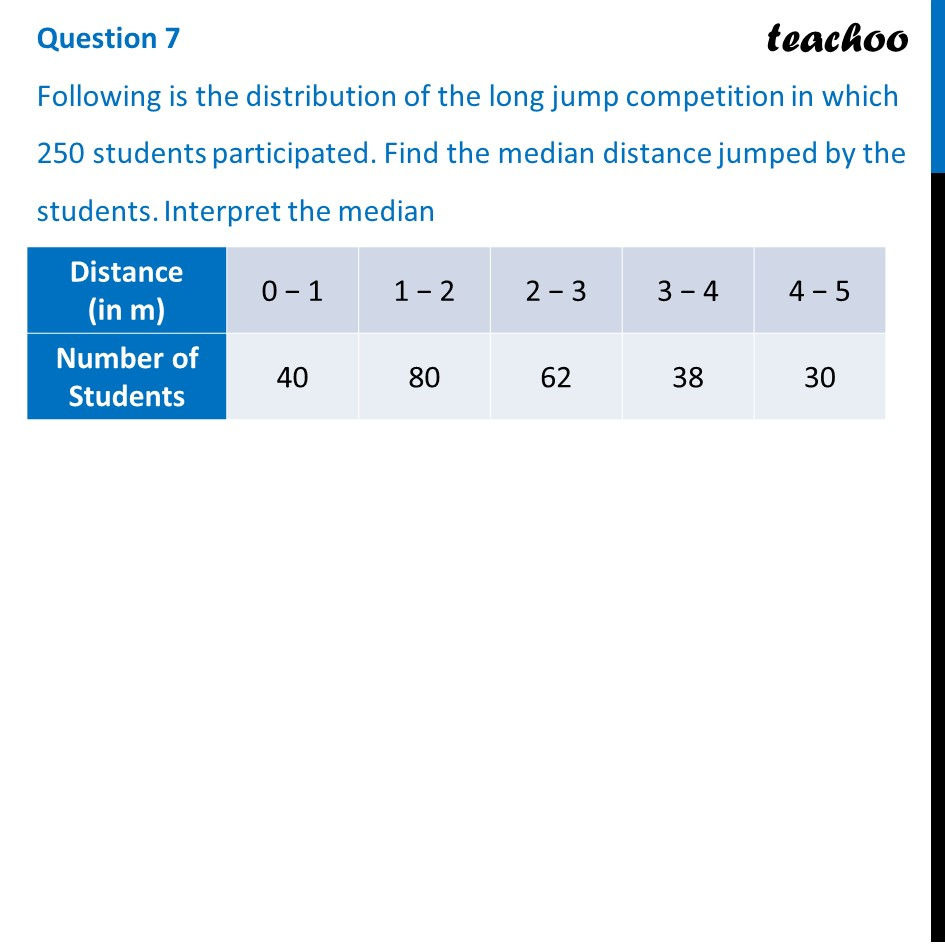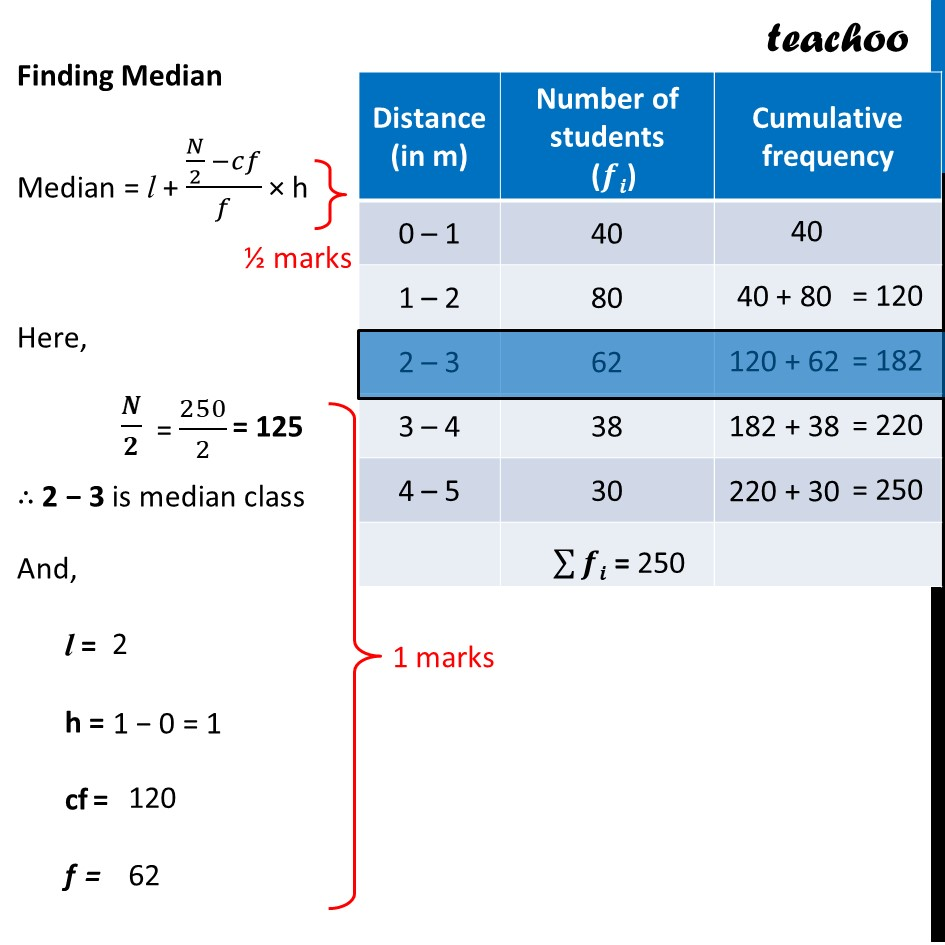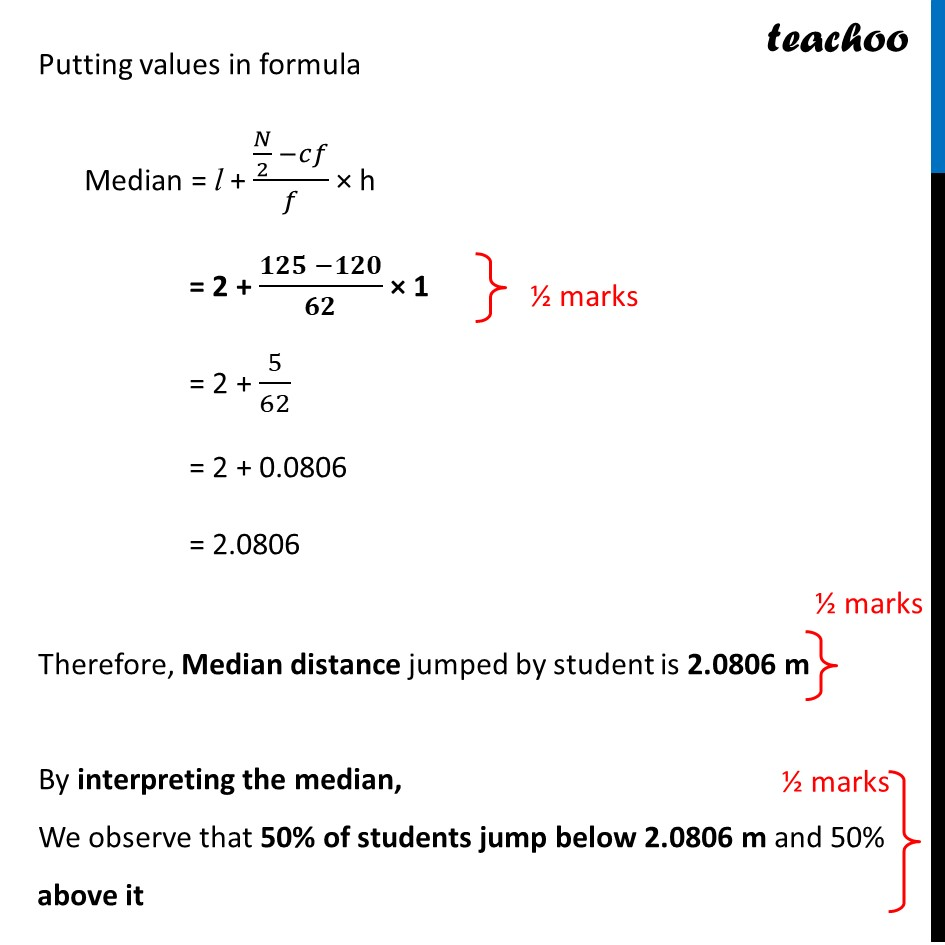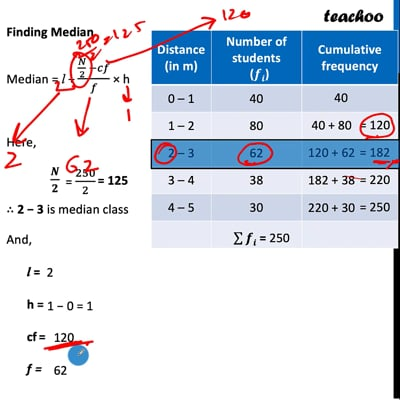CBSE Class 10 Sample Paper for 2022 Boards - Maths Standard [Term 2]

Class 10
Solutions of Sample Papers for Class 10 Boards

## Following is the distribution of the long jump competition in which 250 students participated. Find the median distance jumped by the students. Interpret the median

This question is similar to Ex 14.3, 7 Chapter 14 Class 10 - StatisticsThis video is only available for Teachoo black users

### Transcript

Following is the distribution of the long jump competition in which 250 students participated. Find the median distance jumped by the students. Interpret the median Finding Median Median = l + (𝑁/2 −𝑐𝑓)/𝑓 × h Here, 𝑵/𝟐 ∴ 2 − 3 is median class And, l = h = cf = f = Putting values in formula Median = l + (𝑁/2 −𝑐𝑓)/𝑓 × h = 2 + (𝟏𝟐𝟓 −𝟏𝟐𝟎)/𝟔𝟐 × 1 = 2 + 5/62 = 2 + 0.0806 = 2.0806 Therefore, Median distance jumped by student is 2.0806 m By interpreting the median, We observe that 50% of students jump below 2.0806 m and 50% above it